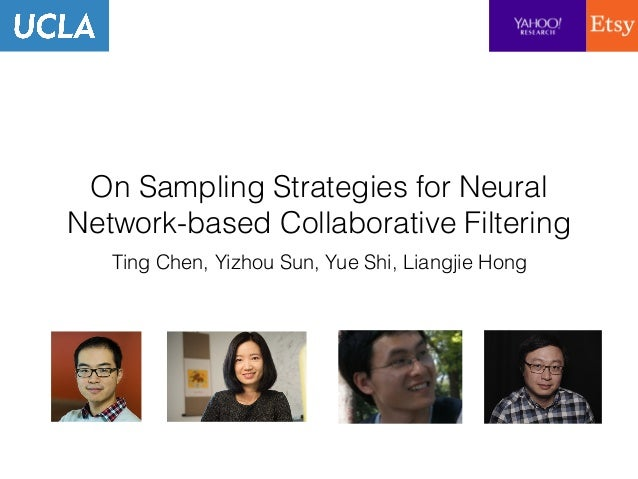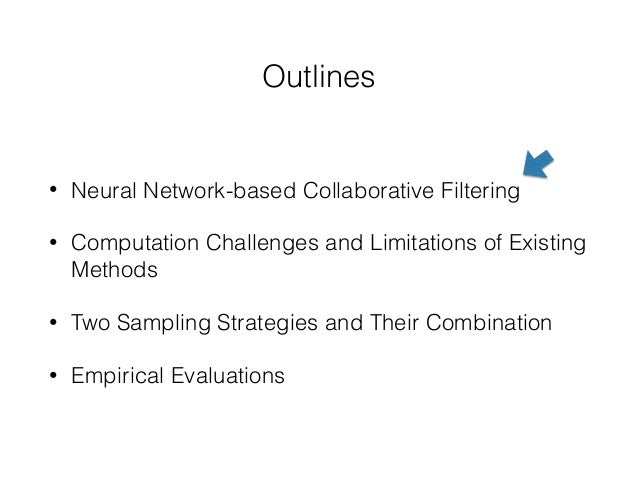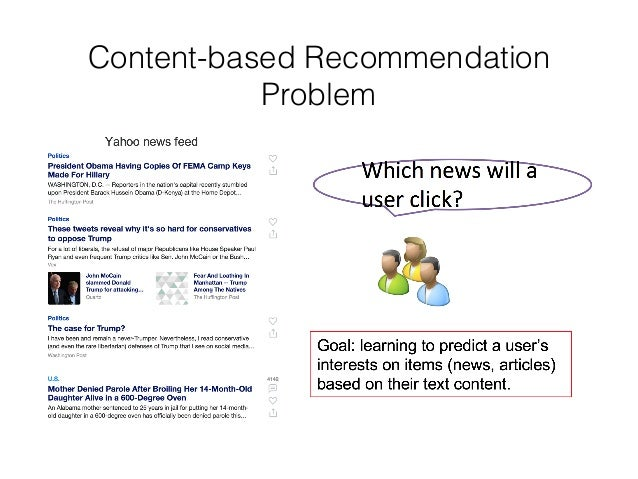Successfully reported this slideshow.Upcoming SlideShare
×

ofUpcoming SlideShare
Next

0 Likes

Share

# On Sampling Strategies for Sampling Strategies-based Collaborative Filtering

paper: http://web.cs.ucla.edu/~yzsun/papers/2017_kdd_sampling.pdf
code: https://github.com/chentingpc/nncf

### Related Books

#### Free with a 30 day trial from Scribd

See all
• Be the first to like this

### On Sampling Strategies for Sampling Strategies-based Collaborative Filtering

1. 1. On Sampling Strategies for Neural Network-based Collaborative Filtering Ting Chen, Yizhou Sun, Yue Shi, Liangjie Hong
2. 2. Outlines • Neural Network-based Collaborative Filtering • Computation Challenges and Limitations of Existing Methods • Two Sampling Strategies and Their Combination • Empirical Evaluations
3. 3. Content-based Recommendation Problem
4. 4. Neural Network-based Collaborative ﬁltering
5. 5. Functional Embedding ruv = f(xu)T g(xv) Embedding functions Interaction function fu, gv 2 RdEmbeddings:
6. 6. • If we have no additional features for users and items (reduced to conventional MF) Embedding Functions • We have text features for items ruv = uT u vv ruv = uT u g(xv) Neural networks Embedding vector uu = f(xu) = WT xu id-based one-hot vector
7. 7. Text Embedding Function g(.) [Y. Kim, AAAI’14] Convolutional Neural Networks Recurrent Neural Networks (LSTM) [Christopher Olah]
8. 8. Implicit Feedbacks and Loss Functions • We deﬁne loss based on implicit feedbacks [Hu’08, Rendle’09] • Interactions are positive • Non-interactions are treated as negative (user, item) as a data point (user, item+, item-) as a data point
9. 9. Training Procedure Have different sampling strategies
10. 10. Outlines • Neural Network-based Collaborative Filtering • Computation Challenges and Limitations of Existing Methods • Two Sampling Strategies and Their Combination • Empirical Evaluations
11. 11. Computation Cost Using Different Embedding Functions Computation cost is dominated by the neural network computation (forward / backward) for items/texts.
12. 12. Major Computation Cost Breakdown User function computation Item function computation Interaction function (dot product) computation tf tg ti 10 100 1 (both forward/backward) Very rough order of magnitude estimate of time units (depending on speciﬁc conﬁgurations)
13. 13. Computation Cost in a Graph View The loss functions are deﬁned over interactions/links, but the major computation burden are on nodes. Pointwise Loss Pairwise Loss
14. 14. Mini-batch Sampling Matters • Since certain data points (links/interactions) share the same computations (on nodes). • Different mini-batch sampling can result in different computations.
15. 15. Existing Mini-batch Sampling Approaches • IID Sampling [Bottou’10] • Draw positive links uniformly at random • Draw negative links according to negative distribution • Negative Sampling [Rendle’09, Mikolov’13] • Draw positive links uniformly at random • Draw k negative links for each positive link by replacing items
16. 16. • Assuming we sample a batch of b positive links, and k negative links for each positive link. Cost Model Analysis for IID and Negative Sampling tf tg ti are unit computation costs for user/item/interaction functions Computation: almost the same
17. 17. Limitations of Existing Approaches • IID sampling assumes computation costs are independent among data points (links). • So the computation cost cannot be amortized, and thus very intensive. • Negative sampling cannot do better since item function computation is the most expensive
18. 18. Outlines • Neural Network-based Collaborative Filtering • Computation Challenges and Limitations of Existing Methods • Two Sampling Strategies and Their Combination • Empirical Evaluations
19. 19. The Proposed Strategies • Strategy one: Stratiﬁed Sampling. • Grouping loss function terms by shared “heavy- lifting” node, i.e. amortized the computation cost • Strategy two: Negative Sharing. • Once a batch of (user, item) tuples are sampled, we add additional links with not much additional costs. • The two strategies can be further combined.
20. 20. Proposed Strategy 1: Stratiﬁed Sampling • Node computation cost can be amortized if we have multiple links sharing the same node when we sample a mini-batch. • That is to group links according to certain “heavy- lifting” nodes (i.e. loss function terms). • We ﬁrst draw items, then draw associated positive and negative links.
21. 21. Proposed Strategy 1: Stratiﬁed Sampling
22. 22. • Assuming we sample a batch of b positive links, and k negative links for each positive link. Cost Model Analysis for Stratiﬁed Sampling tf tg ti are unit computation costs for user/item/interaction functions Speedup: ~(1+k)s times
23. 23. Proposed Strategy 2: Negative Sharing • Interaction computation is much cheaper than (item) node computation (according to our assumption). • Once user/item nodes are given in a batch, adding more links among them may not increase computation cost much. • Only need to draw positive links!
24. 24. Proposed Strategy 2: Negative Sharing Implementation detail: use efﬁcient matrix multiplication operation for complete interactions
25. 25. • Assuming we sample a batch of b positive links, and k negative links for each positive link. Cost Model Analysis for Negative Sharing tf tg ti are unit computation costs for user/item/interaction functions Speedup: (1+k) times Much more negative links
26. 26. Limitations of Both Proposed Strategies • Stratiﬁed sampling: • Cannot work well with ranking-based loss functions • Negative sharing: • Too much negative interactions, diminishing return • Have-your-cake-and-eat-it solution: • Combine both strategies to overcome their shortcomings, while keeping their advantages. • Draw positive links using Stratiﬁed Sampling, generate negative links using Negative Sharing.
27. 27. Proposed Hybrid Strategy: Stratiﬁed Sampling with Batch Sharing
28. 28. • Assuming we sample a batch of b positive links, and k negative links for each positive link. Cost Model Analysis for Stratiﬁed Sampling with Negative Sharing tf tg ti are unit computation costs for user/item/interaction functions Speedup: (1+k)s times Much more negative links
29. 29. Summary of Cost Model Analysis • Computation cost estimation (using b=256, k=20, t_f=10, t_g=100, t_i=1, s=2) • IID sampling: 597k • Negative sampling: 546k • Stratiﬁed sampling (by item): 72k • Negative Sharing: 28k • Stratiﬁed sampling with negative sharing: 16k (all in time units)
30. 30. Convergence Analysis
31. 31. Outlines • Neural Network-based Collaborative Filtering • Computation Challenges and Limitations of Existing Methods • Two Sampling Strategies and Their Combination • Empirical Evaluations
32. 32. Datasets and Setup • We use CiteULike and Yahoo News data sets. • Test data consists of texts never seen before.
33. 33. Speed-up Comparisons Total speedup = speedup per iter * speedup of # iter
34. 34. Recommendation Performance
35. 35. Convergence Curves Converges faster, and performs better!
36. 36. Number of Negative Examples More negative examples helps, with diminishing return.
37. 37. Number of Positive Links per Stratum
38. 38. Conclusions • We propose a functional embedding framework with neural networks for collaborative ﬁltering, which generalizes several STOA models. • We establish the connection between the loss functions and the user-item interaction graph, which introduces computation cost dependency between links (i.e. loss function terms). • Based on the understanding, we propose three novel mini- batch sampling strategies, that speedup model training signiﬁcantly, at the same time improve the performance.
39. 39. Thank You! code is also available @ https://github.com/chentingpc/nncf.

Total views

1,296

On Slideshare

0

From embeds

0

Number of embeds

1

33

Shares

0